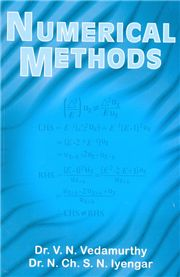# NUMERICAL METHODS V N Vedamurthy & N Ch S N Iyengar-S.CHAND

495.00 445.00

Empirical Laws and Curve Fitting • Theory of Equations • Solution to Numerical, Algebraic and Transcendental Equations • Simultaneous Linear Algebraic Equations • Finite Differences • Interpolation with Equal Intervals • Central Difference Interpolation Formulae • Interpolation with Unequal Intervals • Numerical Differentiation and Integration • Difference Equations • Numerical Solution to Ordinary Differential Equations • Numerical Solution to Partial Differential Equations